# Miscellaneous Exam-Style Questions.

## Problems adapted from questions set for previous Mathematics exams. The questions selected here all contain the text 'Tree Diagram'.

### 1.

GCSE Higher

(a) There are 24 people at a Football Club supporters' meeting in Dudley. Thirteen of them are female.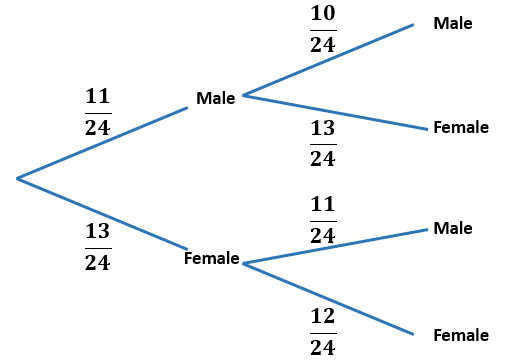First chosen                         Second chosen

Tracey draws the tree diagram above to show how two people could be chosen from the meeting at random. What is wrong with the probabilities shown on the diagram?

(b) Aynuk and Ayli play for Dudley Casuals Football Club. The probabilities that they will score a goal in the next match are 0.2 and 0.35 respectively.

The manager thinks that the probability that both players will score a goal in the next match is 0.2 + 0.35. Is the manager correct? Give reasons for your answer.

### 2.

IB Studies

Neal is attending a Scout jamboree in Japan. He has both boots and trainers to wear. He also has the choice of wearing a cap or not.

The probability Neal wears boots is 0.4. If he wears boots, the probability that he wears a cap is 0.7.

If Neal wears trainers, the probability that he wears a cap is 0.25.

The following tree diagram shows the probabilities for Neal's clothing options at the jamboree.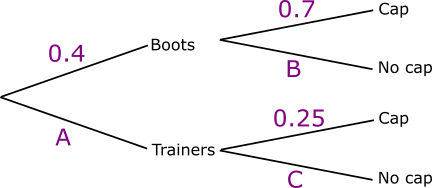(a) Find the value of A.

(b) Find the value of B.

(c) Find the value of C.

(d) Calculate the probability that Neal wears trainers and no cap.

(e) Calculate the probability that Neal wears no cap.

(f) Calculate the probability that Neal wears trainers given that he is not wearing a cap.

(g) Calculate the probability that Neal wears boots on the first two days of the jamboree.

(h) Calculate the probability that Neal wears boots on one of the first two days, and trainers on the other.

### 3.

IB Applications and Interpretation

The Scrumptious Sweet Company sell a variety pack of colourful, shaped sweets. The sweets are produced such that 60% are square and 40% are circular. It is known that 20% of the square shaped sweets and 40% of the circular sweets are coloured red.

(a) Show this information in a tree diagram.

A sweet is selected at random.

(b) Find the probability that the sweet is red.

(c) Given that the sweet is red, find the probability it is circular.

The Scrumptious Sweet Company also produce variety packs of Rainbow Gums. Their specifications state that the colours in each variety pack should be distributed as follows.

 Colour Red Orange Yellow Green Blue Percentage (%) 15 25 15 25 20

Inspector Lou Spowels opens a pack of 90 sweets and records the frequency of each colour:

 Colour Red Orange Yellow Green Blue Observed Frequency 12 21 15 20 22

To investigate if the sample is consistent with the company's specifications, Mr Spowels conducts a $$\chi^2$$ goodness of fit test. The test is carried out at a 5% significance level.

(d) Write down the null hypothesis for this test.

(e) Copy and complete the following table giving the frequencies correct to one decimal place:

 Colour Red Orange Yellow Green Blue Expected Frequency

(f) Write down the number of degrees of freedom.

(g) Find the p-value for the test.

(h) State the conclusion of the test. Give a reason for your answer.

### 4.

IB Studies

Julie chooses a cake from a yellow box on a shelf. The box contains two chocolate cakes and three plain cakes. She eats the cake and chooses another one from the box. The tree diagram below represents the situation with the four possible outcomes where C stands for chocolate cake and P for plain cake.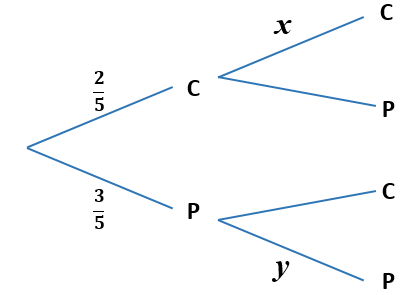(a) Write down the value of $$x$$.

(b) Write down the value of $$y$$.

(c) Find the probability that both cakes are plain.

On another shelf there are two boxes, one red and one green. The red box contains four chocolate cakes and five plain cakes and the green box contains three chocolate cakes and four plain cakes. Ben randomly chooses either the red or the green box and randomly selects a cake.

(d) Copy and complete the tree diagram below.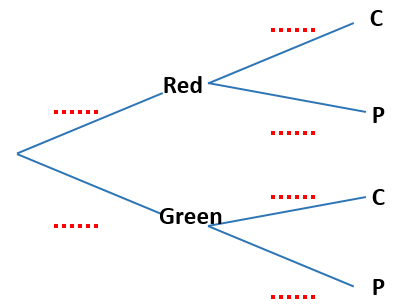(e) Find the probability that he chooses a chocolate cake.

(f) Find the probability that he chooses a cake from the red box given that it is a chocolate cake.

### 5.

IB Standard

Jane and David play two games of golf. The probability that Jane wins the first game is $$\frac56$$. If Jane wins the first game, the probability that she wins the second game is $$\frac67$$.

If Jane loses the first game, the probability that she wins the second game is $$\frac34$$

(a) Copy and complete the following tree diagram.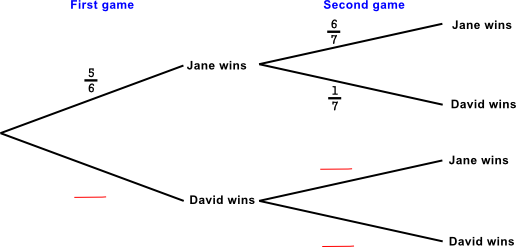(b) Find the probability that Jane wins the first game and David wins the second game.

(c) Find the probability that David wins at least one game.

(d) Given that David wins at least one game, find the probability that he wins both games.

The exam-style questions appearing on this site are based on those set in previous examinations (or sample assessment papers for future examinations) by the major examination boards. The wording, diagrams and figures used in these questions have been changed from the originals so that students can have fresh, relevant problem solving practice even if they have previously worked through the related exam paper.

The solutions to the questions on this website are only available to those who have a Transum Subscription.

Exam-Style Questions Main Page

Search for exam-style questions containing a particular word or phrase:

To search the entire Transum website use the search box in the grey area below.